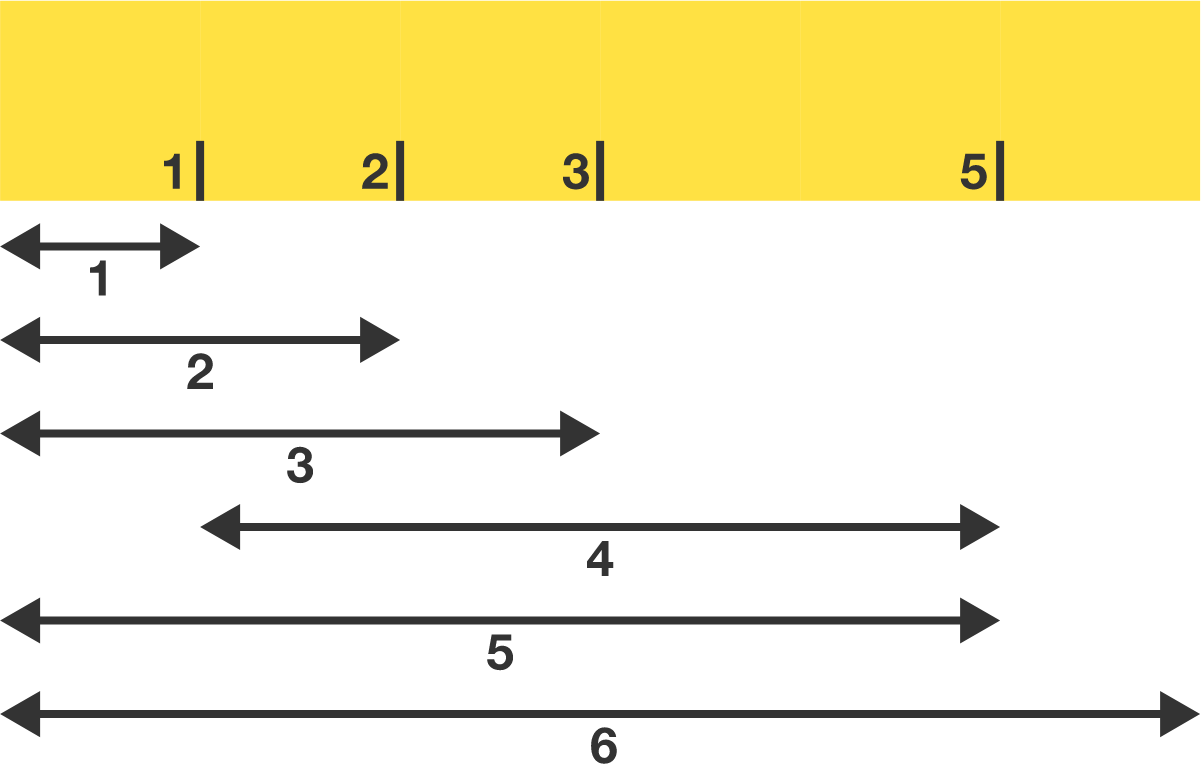# An Old Ruler

Geometry Level 2

Chris has an old 6-inch ruler that is missing the $4 \text{ in}$ mark. However, he can still measure all integer lengths from $1 \text{ in}$ to $6 \text{ in}.$ He'd like to see if he can erase more marks and still be able to measure all integer lengths from $1 \text{ in}$ to $6 \text{ in}.$

What is the minimum number of marks the ruler could have?Chris' ruler can measure all integer lengths from $1 \text{ in}$ to $6 \text{ in}$ with only 4 marks.

Note that all measurements must be made directly using the ends of the ruler and/or its marks. For example, you can't repeatedly use a distance of $1 \text{ in}$ to make larger distances.

×